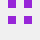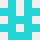# 如何在 LUA 中重定向所有类调用？

``````MyClass.OriginalMyFunction = MyClass.MyFunction -- 存储旧函数

function MyClass:MyFunction(args)
--执行之前做一些事情
MyClass:OriginalMyFunction(args) --使用相同的参数调用原始函数
end
``````stackoverflow用户4070330

``````local MyClass = {}
function MyClass:new()
local newObj = {
x = 0
}
self.__index = self
return setmetatable(newObj, self)
end

function MyClass:get_x()
print("hello from get_x")
return self.x
end

local HookedMyClass = {}

function HookedMyClass:new()
local t = MyClass:new()
local mt = {
__index = function(table, key)
print("hook")
return(MyClass[key])
end
}
return setmetatable(t, mt)
end

local hooked = HookedMyClass:new()

print(hooked:get_x())
``````
2022-02-28 13:14:12stackoverflow用户7504558

``````function new (parent)
local child =  {}
for k,v in pairs(parent) do
if type(v)=="function" then
child[k] = function(...)
print("inherit:")
return v(...)
end
else child[k] = v
end
end
return child
end

my_class = new(class)

class.bar("arg1","arg2")
my_class.bar("arg1","arg2")
print(class.a)
print(my_class.a)
``````

``````bar arg1 arg2
inherit:
bar arg1 arg2
value
value
``````
2022-02-28 14:01:47